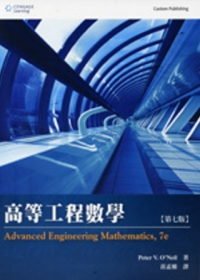{itemname} {itemname}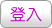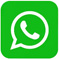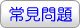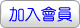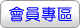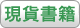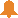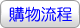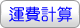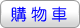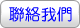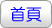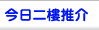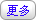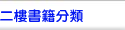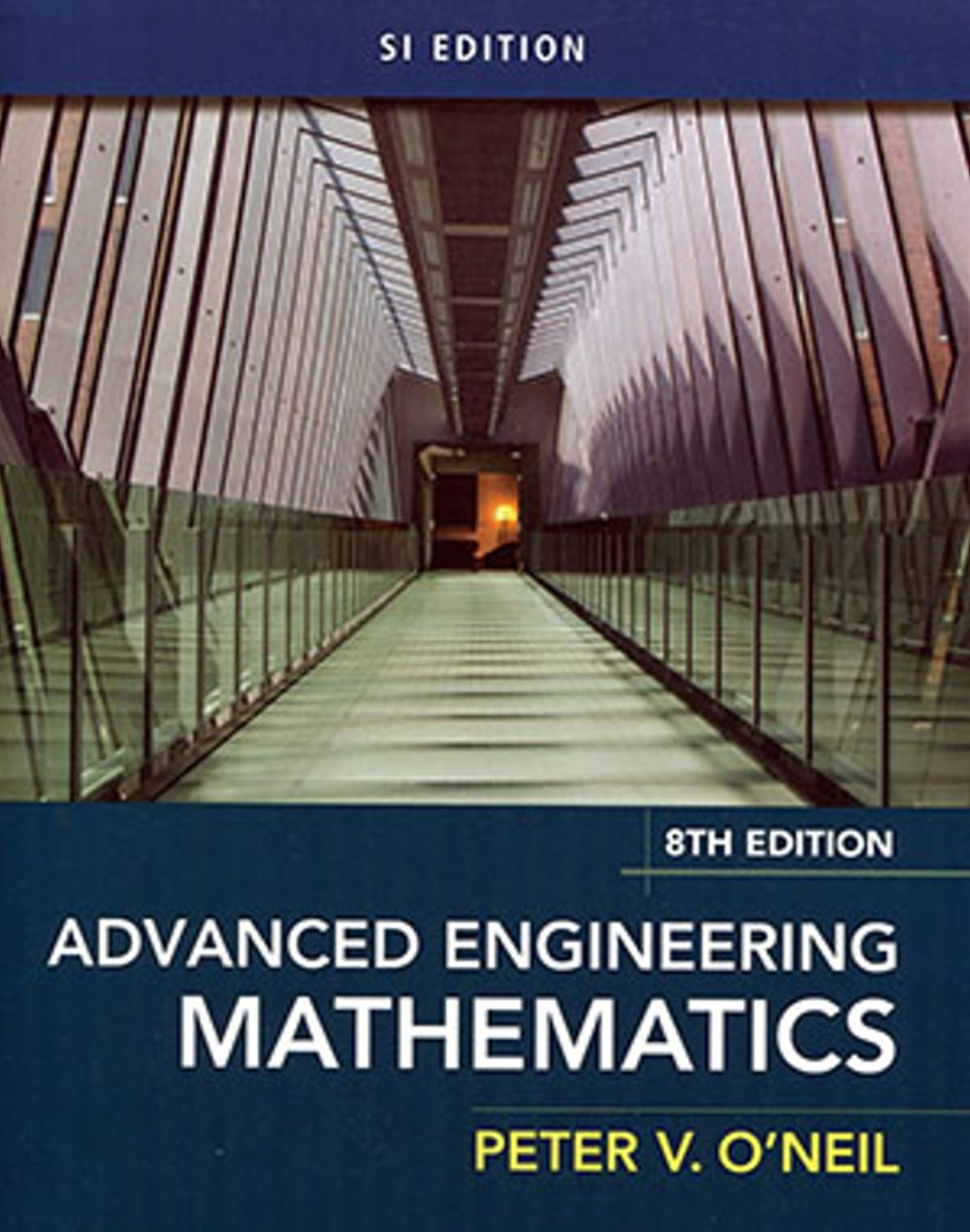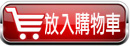沒有庫存 訂購需時10-14天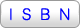9781337274524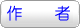Peter V. O’Neil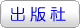華泰文化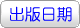2017年6月08日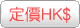473.00  元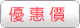HK\$ 449.35省下 \$23.65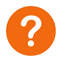ISBN：9781337274524
• 叢書系列：工程數學
• 規格：平裝 / 839頁 / 20.3 x 25 x 2.9 cm / 普通級
工程數學

• 專業/教科書/政府出版品 > 工程類 > 土木工程

Now you can make rigorous mathematical topics accessible to your students by emphasizing visuals, numerous examples, and interesting mathematical models with ONeil’s Advanced Engineering Mathematics. New "Math in Context" broadens the engineering connections for your students by clearly demonstrating how mathematical concepts are applied to current engineering problems. You have the flexibility to select additional topics that are best for your individual course.

PART I: ORDINARY DIFFERENTIAL EQUATIONS?

Ch 1 First-Order Differential Equations?

Ch 2 Second-Order Differential Equations

Ch 3 The Laplace Transform?

Ch 4 Sturm-Liouville Problems and Eigenfunction Expansions?

PART II: PARTIAL DIFFERENTIAL EQUATIONS?

Ch 5 The Heat Equation?

Ch 6 The Wave Equation?

Ch 7 Laplace’s Equation?

Ch 8 Special Functions and Applications?

Ch 9 Transform Methods of Solution?

PART III: MATRICES AND LINEAR ALGEBRA?

Ch10 Vectors and the Vector Space Rn?

Ch11 Matrices, Determinants, and Linear Systems?

Ch12 Eigenvalues, Diagonalization, and Special Matrices?

PART IV: SYSTEMS DIFFERENTIAL EQUATIONS?

Ch13 Systems of Linear Differential Equations

Ch14 Nonlinear Systems and Qualitative Analysis?

PART V: VECTOR ANALYSIS?

Ch15 Vector Differential Calculus?

Ch16 Vector Integral Calculus?

PART VI: FOURIER ANALYSIS?

Ch17 Fourier Series?

Ch18 Fourier Transforms?

Ch19 Complex Numbers and Functions?

Ch20 Integration?

Ch21 Series Representations of Functions?

Ch22 Singularities and the Residue theorem?

Ch23 Conformal Mappings

 其 他 著 作 1. 工程數學(8版)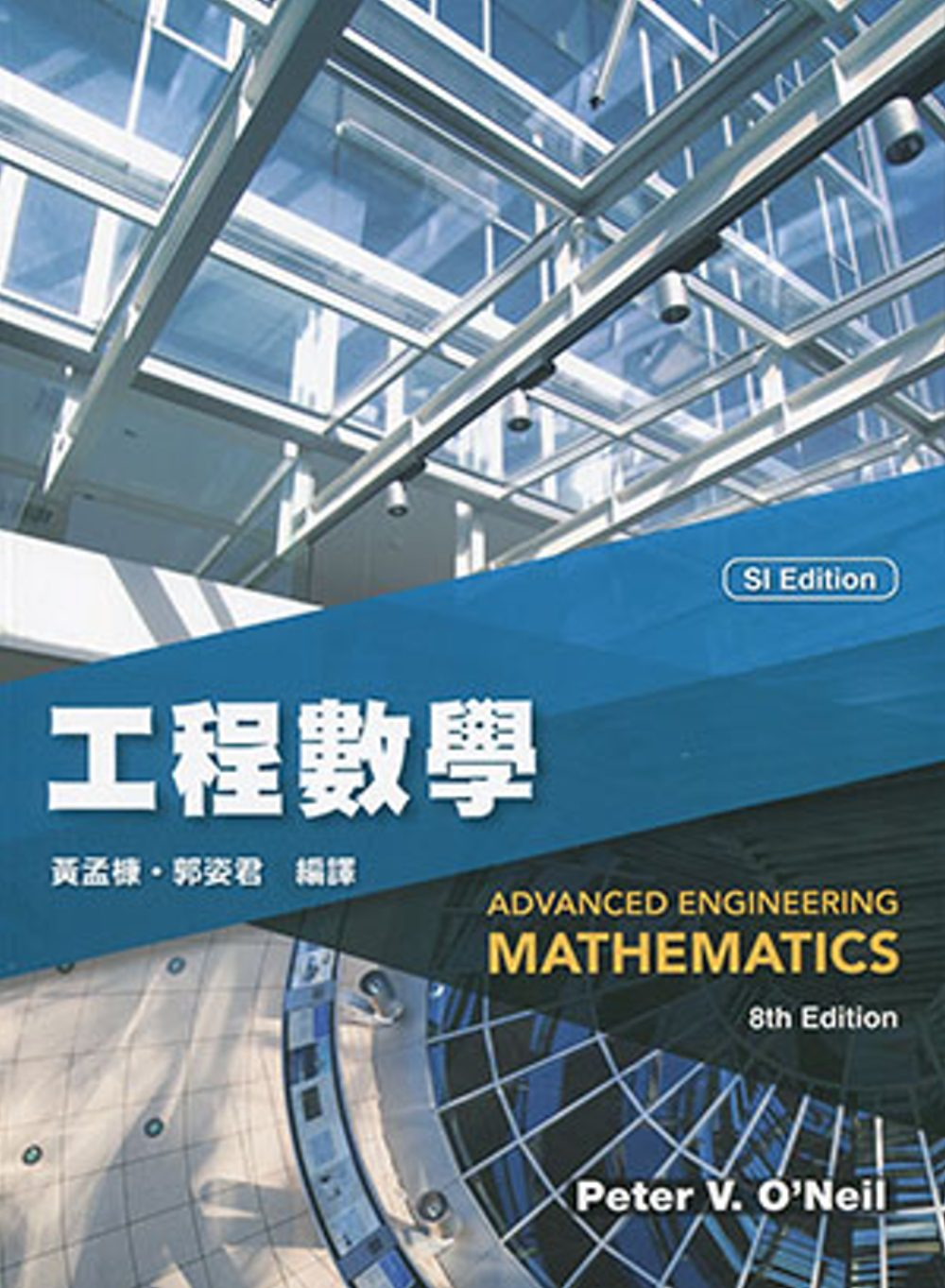2. 高等工程數學(8版)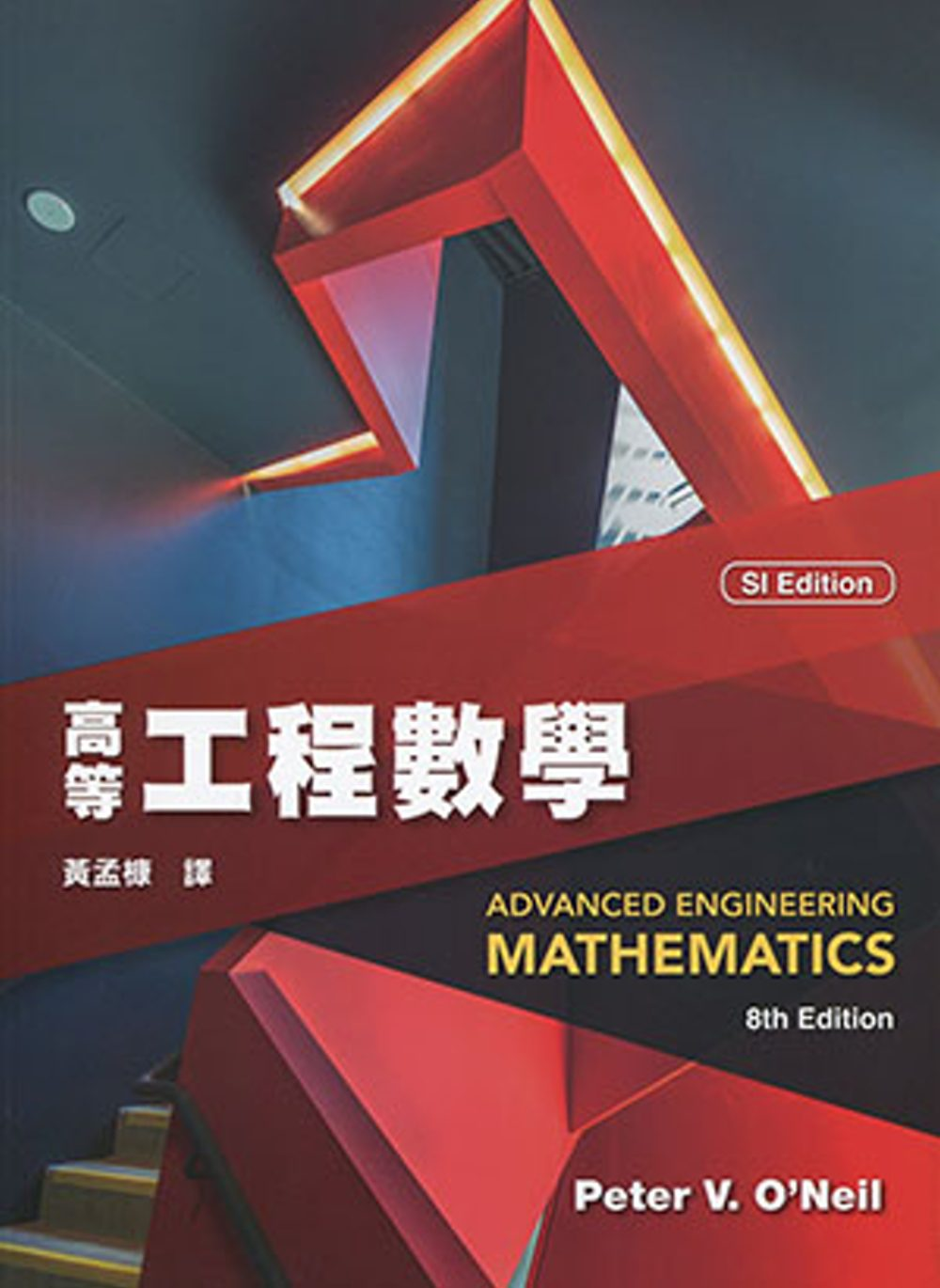3. Advanced Engineering Mathematics(SI Edition)(Custom Solutions)(8版)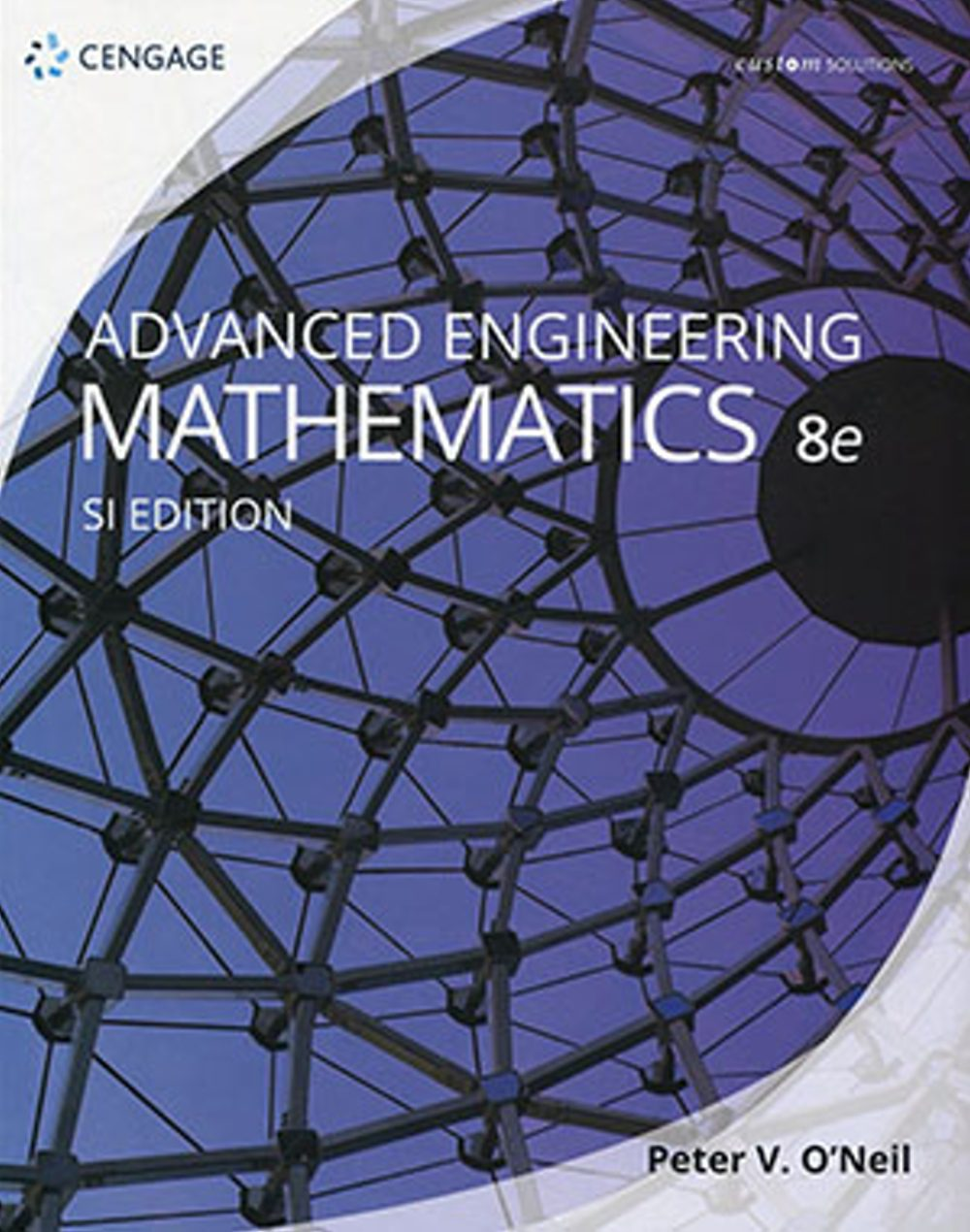4. Advanced Engineering Mathematics (Custom Edition) (bundle Coursemate)7/E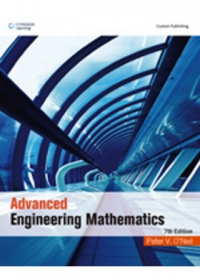5. 高等工程數學(7版) O’Neil/ Advanced Engineering Mathematics 7/e